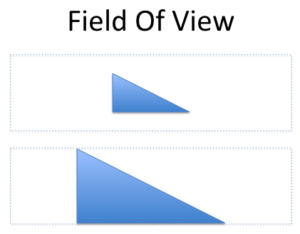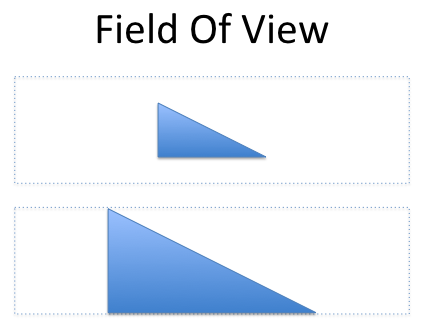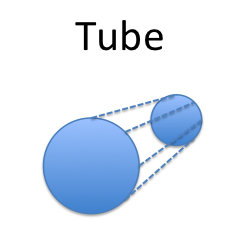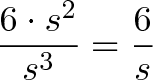- BetterExplained - https://betterexplained.com -

# Understanding Why Similarity WorksSimilarity has bothered me for a long time. Why do all circles have the same formula for area — how do we know nothing sneaky happens when we make them larger? In physics, don’t weird things happen when you scale things (particles, insects, small children) to gargantuan sizes? You’re saying that every circle has the same formula, yet a 300-foot honeybee cannot fly?

Here’s the deal:

• Similar shapes are zoomed versions of each other. Because we can’t tell them apart (read on for why), they must have the same internal formulas for area, perimeter, and so on.

• However, items with the same formula aren’t exchangeable! Sure, all humans (from children to NBA players) have the “formula” that armspan = height. Fine — does that mean a 7 foot Sasquatch and my 18-inch nephew are equally good basketball players?

My “aha!” moment was separating the common formula (armspan = height) from the applicability of individual instances (infant vs. Sasquatch).

## Why Scaled Objects Have The Same Formula

Thought experiments helped me realize that absolute size doesn’t matter when figuring out whether a formula can hold for all instances of a shape. An insight is that perceptions of “size” are often determined by us, the observer, and not the shape itself.

Field of Vision

Imagine a triangle on a piece of paper. It takes up some amount of room in your field of vision — say, 30%.

Now, move closer to the paper, so the triangle takes up most of your view — say, 90%. What changed? The triangle is the same, but the sides appear much bigger. Yet we know that the core properties (area, perimeter, etc.) haven’t changed — otherwise, we’d need to know someone’s distance from a triangle when calculating area!Create a Tube

This time, imagine a paper circle. Now, thicken the paper until you make a cylinder (of equal diameter) extending into the distant horizon. The part of the cylinder a few feet off “looks” to be 1/2 the size of the disc before you, yet you know they are the same size. The ratios inside (circumference to diameter, area to radius, etc.) must be the same also.Photoshop Zoom

Imagine a triangle on a computer screen. Make all sorts of formulas for area, perimeter, and so on. Now, zoom the triangle by 300% and measure again. What changed? Sure, everything was bigger on the second measurement, but does the triangle “know” it’s being zoomed and change itself to make the formulas different?

Measurement Unit

Suppose you’re measuring ratios on a shape with your trusty ruler. You have your table full of figures: area to perimeter, diagonal to side, and so on. But whoops! It looks like you had written “cm” when you were actually measuring the sides in inches.

Do you need to redo your table because you were using the wrong unit? Does the shape know what units you’ve been using?

## Enter Physics

My conundrum started when remembering a factoid from biology class: Godzilla couldn’t exist because he would overheat. Big lizards can’t do the same things little lizards can.

Why?

For simplicity, let’s assume Godzilla is a lizard cube. For any cube of side “s”, the volume is s3, and the surface area is 6 * s2.

Now, let’s assume that heat generated is proportional to volume (essentially your mass), and cooling is proportional to surface area (amount of skin you have exposed to that cool, breezy air). How much cooling do you have for each unit of girth mass?$\displaystyle{\frac{6 \cdot s^2}{s^3} = \frac{6}{s}}$

For every unit of volume, we have 6 / s “surface area units” available to cool it. If s = 1cm (for example), then we have 6 / 1 = 6 square centimeters to cool ourselves for each cm3 of volume. Great.

But what if s = 10cm? Uh oh. Now we have 6 / 10 = .6 square centimeters of cooling. And if s = 100cm we only have .06 sq cm of cooling. At some point, our cooling cannot balance our heat requirements and Godzilla falls over. (Suppose he needs at least .1 square cm of cooling for each cubic cm to stay alive).

Remember our insight:

• s3, 6 * s2, and 6 / s are common patterns in all cubes, no matter the size
• 6 / 1 = 6, 6 / 10 = .6, 6 / 100 = .06 are particular instances of the “surface area to volume” formula. Some meet our heat requirements, others don’t.

If you’re interested, there are other structural problems with a lizard of that magnitude.

## Examples Abound

The idea of finding patterns in similar shapes (and separating them from specific examples) is ubiquitous in math and the sciences. Here’s a few examples of “similarity” which often aren’t labeled as such.

Discovering Pi

Pi is the most famous example of similarity — all circles share the same ratio (circumference / diameter = pi). Again, no matter how much we zoom to make one circle appear like another, every circle has this fundamental trait.

The Physics of Spheres

A sphere is the most space-efficient shape — it gives the most volume for the least surface area. No matter if you’re an elephant or mouse, you’ll conserve the most heat by curling into a ball.

Planets and raindrops are spherical because of these unique properties — even though the scale difference for each example is enormous.

Trigonometry

Sine, cosine, and the rest of the trig family work off angles. And angles are perfect for similarity since size doesn’t matter (how long do the sides of a 45 degree angle need to be? It doesn’t matter!).

Because triangles with the same angles are similar, we can use the ratios inside one (i.e, triangles that fit inside the unit circle) and scale up the result for any example we need.

Algorithm Running Time

The running time of algorithms [O(n), O(n*log(n)), O(n2), etc.] are based on finding a “similarity class” describing the runtime. An algorithm with O(n2) should run 4x as slowly when the number of inputs are doubled.

However, for specific instances, the desired algorithm may be different: running 10 inputs with a O(n3) algorithm can be faster than running 10 million inputs with an O(n).

Object Oriented Programming

In programming, members of the same class (“similarity” class!) may share formulas like $Area = \pi r^2$. However, each instance of that class may have a different value of “r”. The class provides the general patterns while the instances provide the details.

## Closing Thoughts

A few observations:

• Separate the common formula from particular instances of a shape. All circles are similar, but a bigger pizza is better than small one, right?
• Analogies help us remember. I have silly reminders about infant NBA players and “Godzilla cubes” that makes the “pattern vs example” concept more clear.
• The idea of similarity is broader than just geometry — it’s about identifying classes of items that share the same internal properties.

The actual definition of similarity is more nuanced; you can reverse it and say shapes are similar if formulas based on their distance are always the same (they are uniformly scaled or dilated). But, those are fun diversions for another day — happy math!